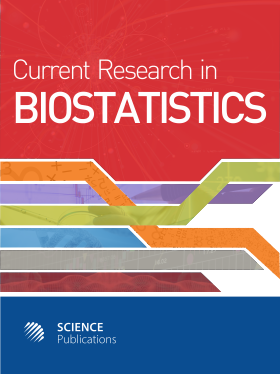Frequency: Continuous
ISSN: 2524-2210 (Print)
ISSN: 2524-2229 (Online)
Research Article Open Access

# COMPARISON OF FIVE EXACT CONFIDENCE INTERVALS FOR THE BINOMIAL PROPORTION

Iori Sakakibara1, Emiko Haramo1, Akimasa Muto1, Ikuya Miyajima1 and Yohei Kawasaki2
• 1 , Japan
• 2 Tokyo University of Science, Japan
Current Research in Biostatistics
Volume 4 No. 1, 2014, 11-20

DOI:

Submitted On: 24 June 2014 Published On: 18 July 2014

How to Cite: Sakakibara, I., Haramo, E., Muto, A., Miyajima, I. & Kawasaki, Y. (2014). COMPARISON OF FIVE EXACT CONFIDENCE INTERVALS FOR THE BINOMIAL PROPORTION. Current Research in Biostatistics, 4(1), 11-20. https://doi.org/10.3844/amjbsp.2014.11.20

## Abstract

The Wald interval is easy to calculate; it is often used as the confidence interval for binomial proportions. However, when using this confidence interval, the actual coverage probability often falls under the nominal coverage probability in small cases. On the other hand, several confidence intervals where the actual cover age probability does not fall under the nominal coverage probability are suggested. In this study, we intro-duce five exact confidence intervals where the actual coverage probability does not fall under the nominal coverage probability and we calculate the expected length of the confidence intervals and compare/verify the accuracy of the coverage probabilities. Further, we examined the characteristics of these five exact confidence intervals at length. Coverage probability of Sterne was significantly closer to 0.95 than the other confidence intervals and stable. Its expected Length are not scattered in the width compared with the other methods. As a result, we found that the quality of the confidence interval based on the Sterne test is its availability for small samples.

• 1,706 Views